### ZKSwap团队深入解读零知识证明算法之Zk-stark（三）：Low Degree Testing

#### 新的实例

Alice Claim：“我有 1000000 个数，他们都在 [0,9] 范围内”。为了方便验证者 Bob 验证，Alice 首先要对 Claim 进行 Arithmetization 转换。过程如下图 1 所示(图中：黑色箭头代表主流程，红色箭头代表附加说明信息，黄色圈对应下面详细说明的索引)。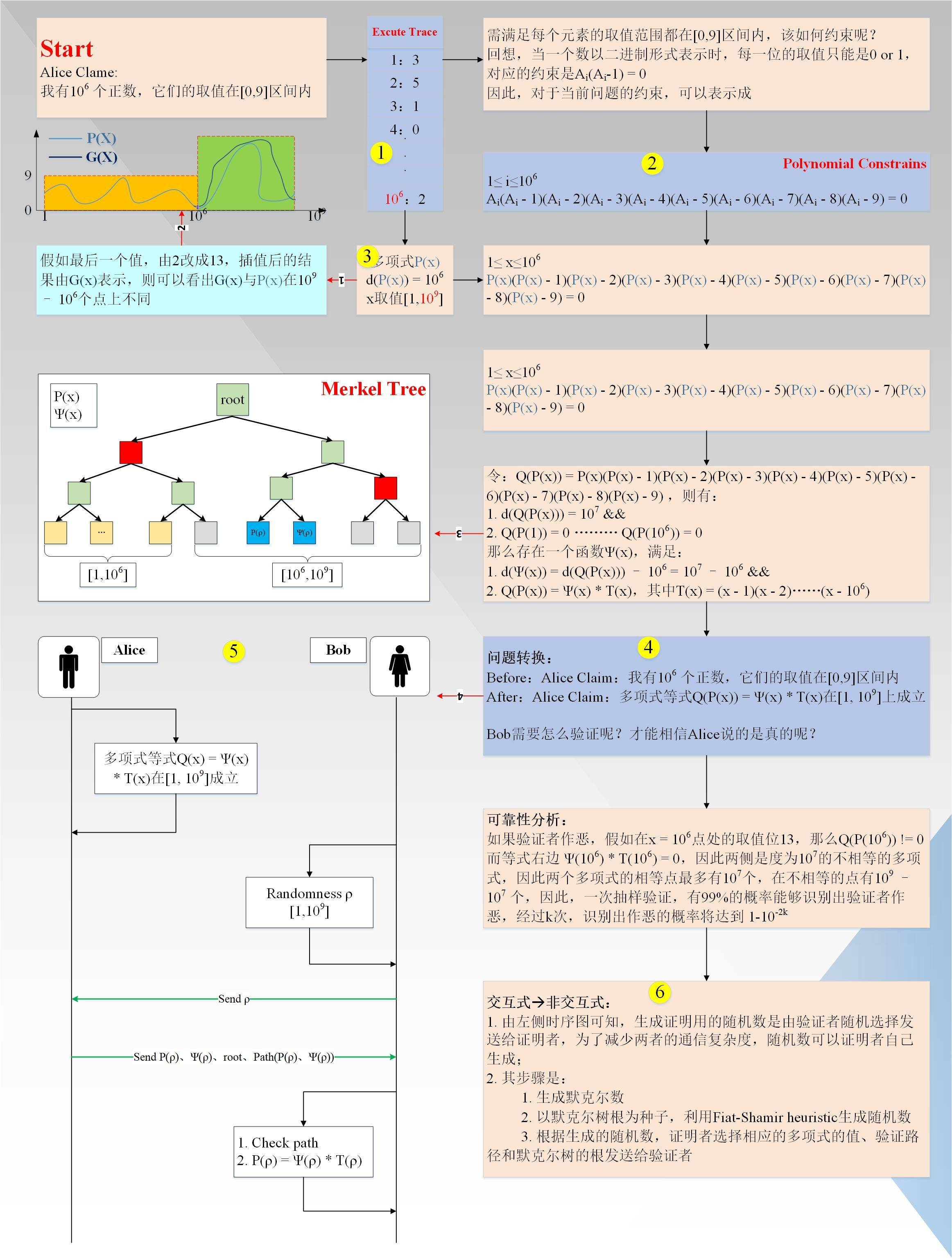1. 首先生成执行轨迹(EXCUTE TRACE)，事实上，它是一张表，总共有 1000000 行（实际上，为了达到零知识的目的，还需要在执行轨迹后面增加一些随机值，具体数量是由证明者和验证者统一协调决定的，作为一个扩展，不具体讲述）；
2. 生成多项式约束(Polynomial Constrains)，多项式约束满足执行轨迹的每一行(个人理解：步骤 1，2 没有一定的先后依赖关系，只是习惯上先生成执行轨迹，再生成约束多项式)；
3. 对执行轨迹进行插值，得到一个度小于 1000000 的多项式 P(x)、x 取值[1,1000,000]，并计算更多点上的值，x 取值范围扩大到11000000000；假如，证明者有一个值不在[0,9] 范围内(图中红线 1/2 所示)，假如就是第 1000000个点，它实际的值是 13，大于 9，其插值后的曲线 G(x) 如图所示，图中 P(x) 为有效曲线，G(x) 为无效曲线。可以看出，两条曲线在变量 x 取值 [1,1000000000] 范围内，最多有 1000000个交点，即有1000000000 - 1000000 个点不同，这很重要。
4. 将插值后的多项式 P(x) 和多项式约束进行组合变换，最终得到的形式为：

Q(P(x)) = Ψ(x) * T(x)，其中 T(x) = (x - 1)(x - 2)……(x - 1000000)，x 取值[1,1000000000]

1. 至此，问题就转化成了，Alice 宣称“多项式等式在变量x取值[1,1000000000]范围内成立”的问题。那么验证者 Bob 该如何验证呢？具体过程如下（图中红线 3/4 所示）： a. 证明者 Alice 在本地计算多项式P(x)、Ψ(x)在所有点上的取值，对！从1至1000000000，并形成一个默克尔树； b. 验证者 Bob 随机的从[1,1000,000000]内选取一个值 ρ，并发送给证明者Alice，要求其返回对应的信息（事实上为了达到零知识的目的，只允许从[1000,000,1000,000,000]上随机选择一点）； c. 证明者 Alice 返回 P(ρ)、Ψ(ρ)、root、AuthorizedPath(P(ρ)、Ψ(ρ))给验证者Bob； d. 验证者Bob首先根据默克尔树验证路径验证值 P(ρ)、Ψ(ρ)的有效性，然后等式Q(P(ρ)) = Ψ(ρ) * T(ρ)，如果成立，则验证通过；

• 上述的验证过程为交互式的，如果是非交互式的，可以利 Fiat-Shamir heuristic 进行变换，以默克尔树的根作为随机源，生成要查询的随机点；

#### LDT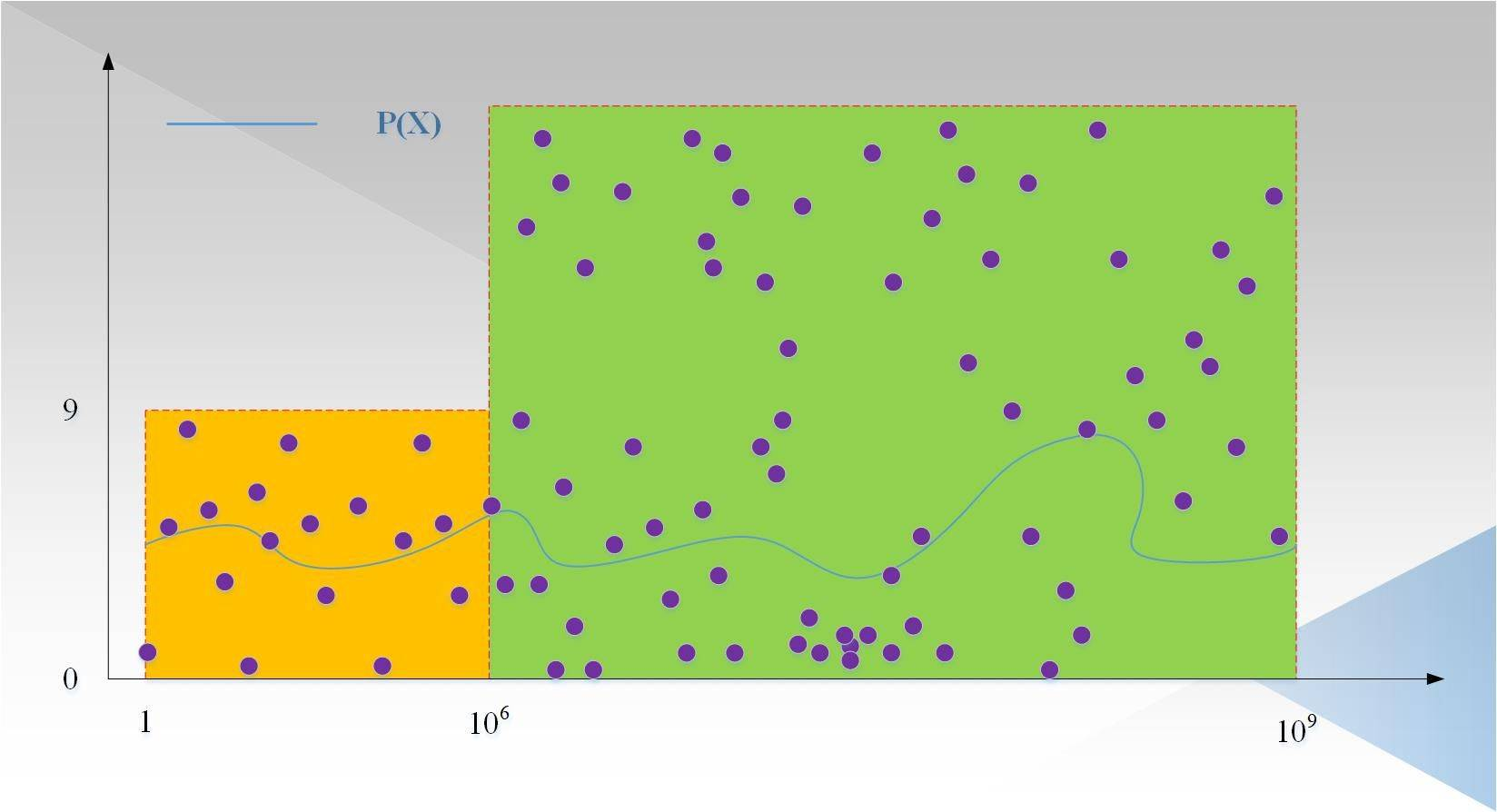1. 验证者 Bob 随机选取三个值 a,b,c，发送给证明者 Alice；
2. 证明者 Alice 返回 f(a),f(b),f(c)；
3. 验证者 Bob 插值出度小于 3 的多项式 g(x)，然后再随机选取一个点 d，发送给证明者；
4. 证明者 Alice 返回f(d)；
5. 验证者 Bob 比对 f(d)和g(d)的值，如果相等，则证明成立。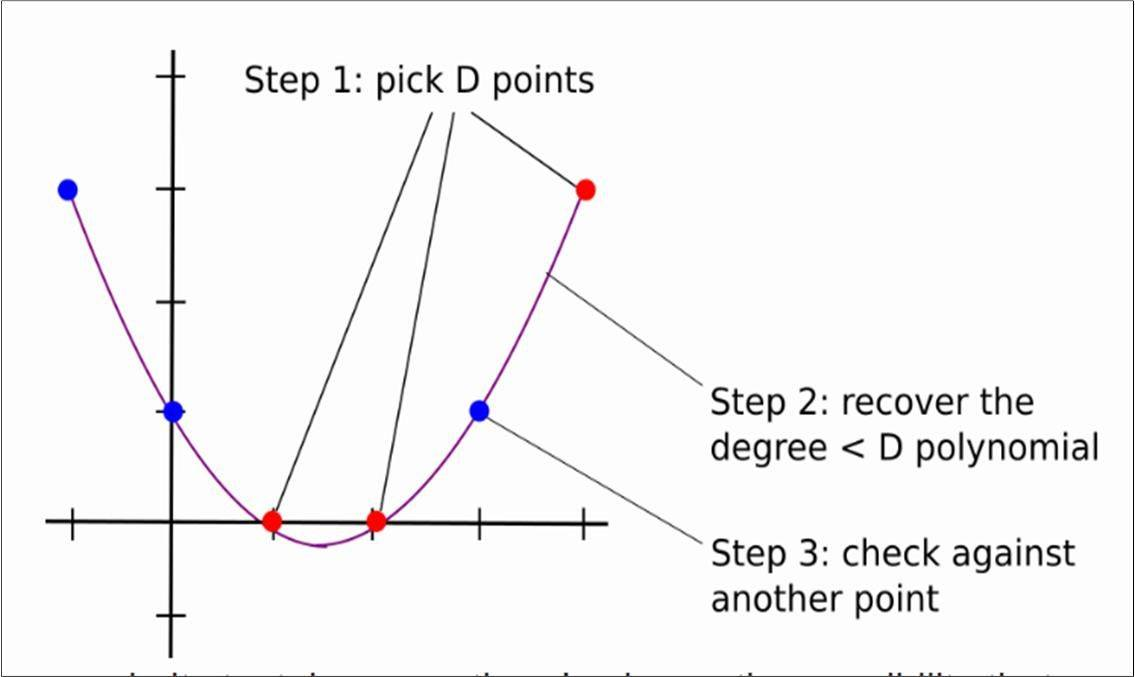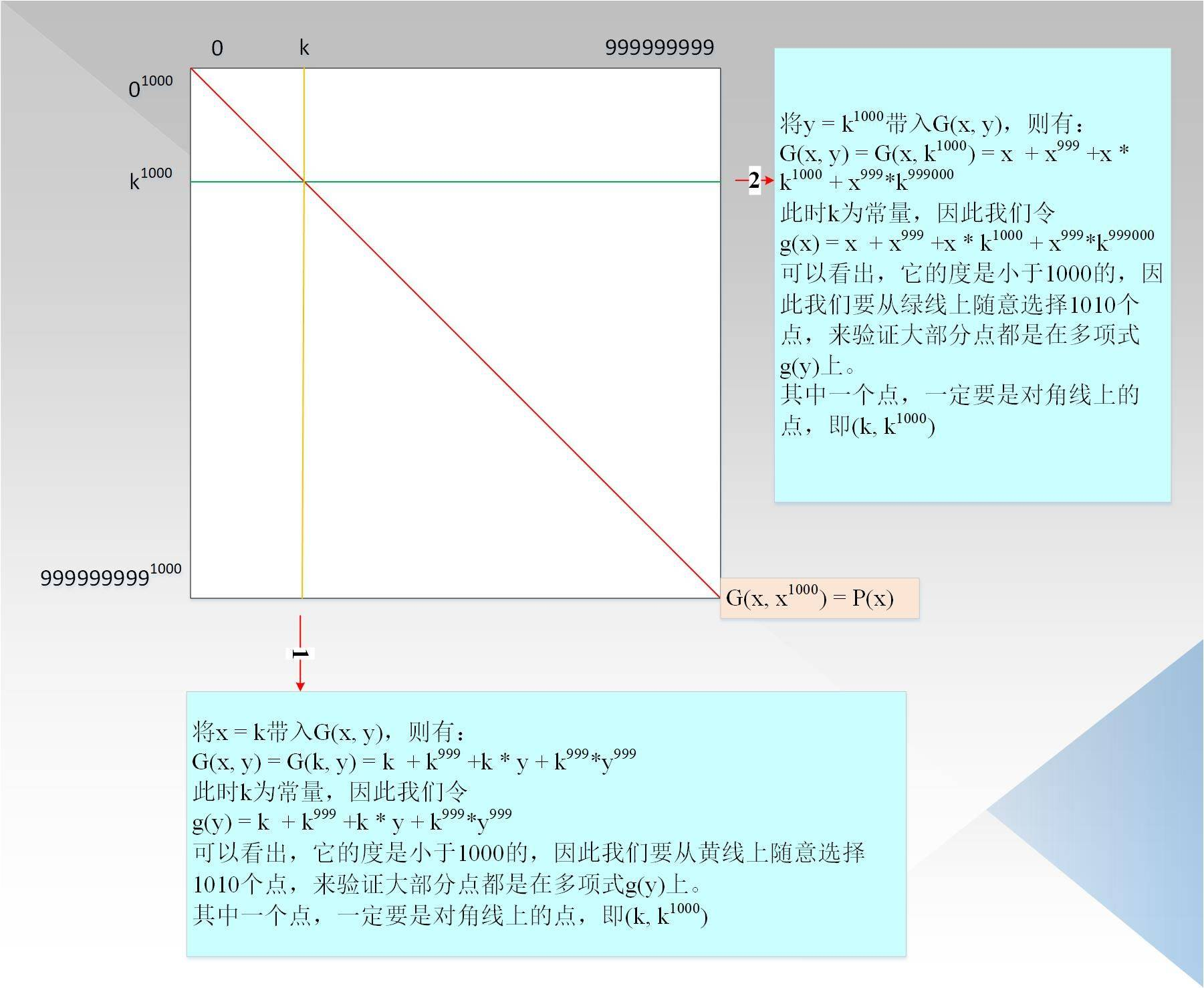P(x) = x + x^999 + x1001 + x^999999 ，而实际的 P(x)，我们在这里标记为 P`(x) ，其形式是：

P`(x) = x + x^999 + x^1001 + x^1010999

• 虽然上述实现了在交互次数小于 D 的情况下，完整 LDT 验证，但是证明者的复杂度过于庞大，至少 10^18 的复杂度远远大于原始的计算，因此需要一些优化方案，降低复杂度。话不多说，直接引入有限域，毕竟在实际项目中，我们可不希望数值本身过于庞大。直接引用费马小定理的结论：在有限域p内，如果满足(p - 1) 能被 k 整除，则映射 x => x^k的像只有(p -1) / k + 1个。下图 5 以 p = 17，映射 x=> x^2 为例：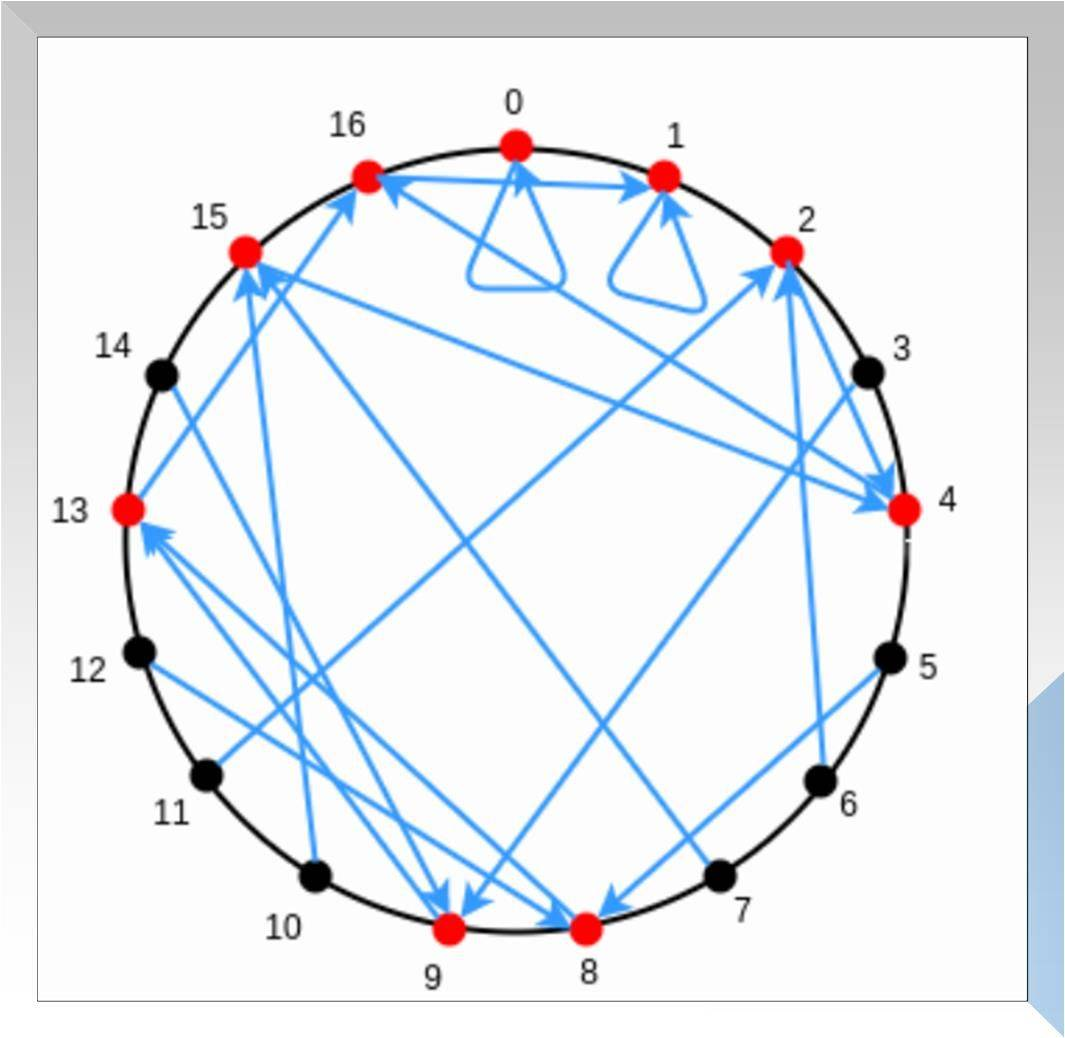• 为素数
• p - 1 能被 1000 整除
• p 要大于 10^9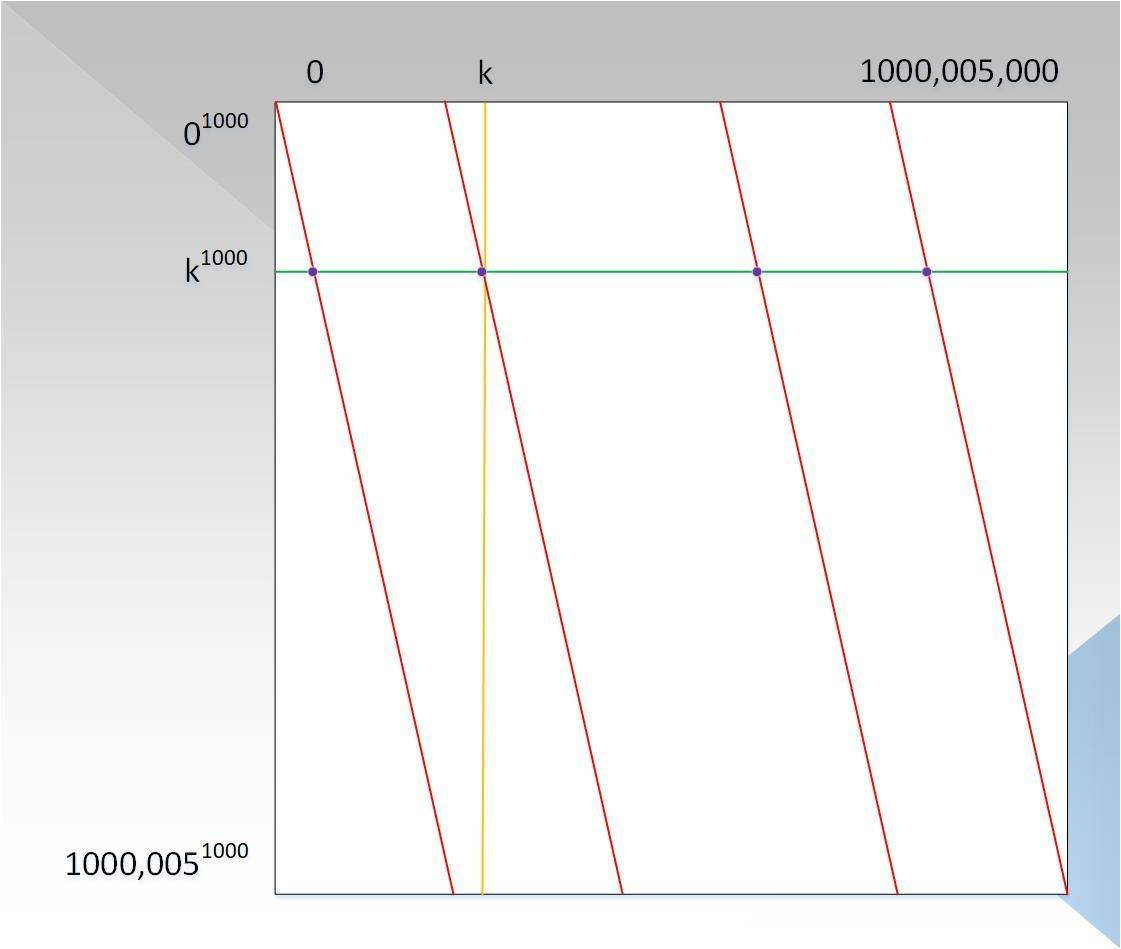1. 还能不能继续优化呢？答案是肯定的。回想起前面所述的验证过程，对于每一行/列，验证者都要获取 1000 个点进行插值得出一个度小于 1000 的多项式，仔细观察图 6，对于每一行，原始数据里不就是有 1000 个数么？那我们干脆选这些点插值出一个度小于1000 的多项式，然后只需要随机让证明者再计算任何一列，并且证明沿着列上的点都在度小于 1000 的多项式上，并且列上的点也在对应的利用原始数据插值出的行多项式上。此时，证明者复杂度从 10^15 减少到了 10^9 次方。
2. 总结：个人理解，从步骤 1 到步骤 5，其实是 PCP 到 IOP 的选择过程。 a. PCP 要求证明者生成全部的证据，然后验证者多次随机选取其中的某一部分进行验证，但是这样，证明者的复杂度仍然很高； b. IOP 要求证明者不用生成全部的证据，根据多次的交互，每次生成只需生成部分证据，使得证明的复杂度和 D 呈近似线性关系；
3. 证明者复杂度已经降低到了与 D 呈拟线性关系，验证者的复杂度虽然是亚线性，交互次数已经低于 D，但是能不能优化到更低呢？基于证明复杂度的最优设置，我们继续探索验证复杂度的优化之路，回顾 P(x) = x + x^999 + x^1001 + x^999999 = x + x(x^2)^499 + x(x^2)^500 + x(x^2)499999，令G(x, y) = x + xy^499 + xy^500 + xy^499999，则当 y = x^2时，有 G(x, y) = G(x, x^2) = x + x(x^2)^499 + x(x^2)^500 + x(x^2)*499999 = P(x)。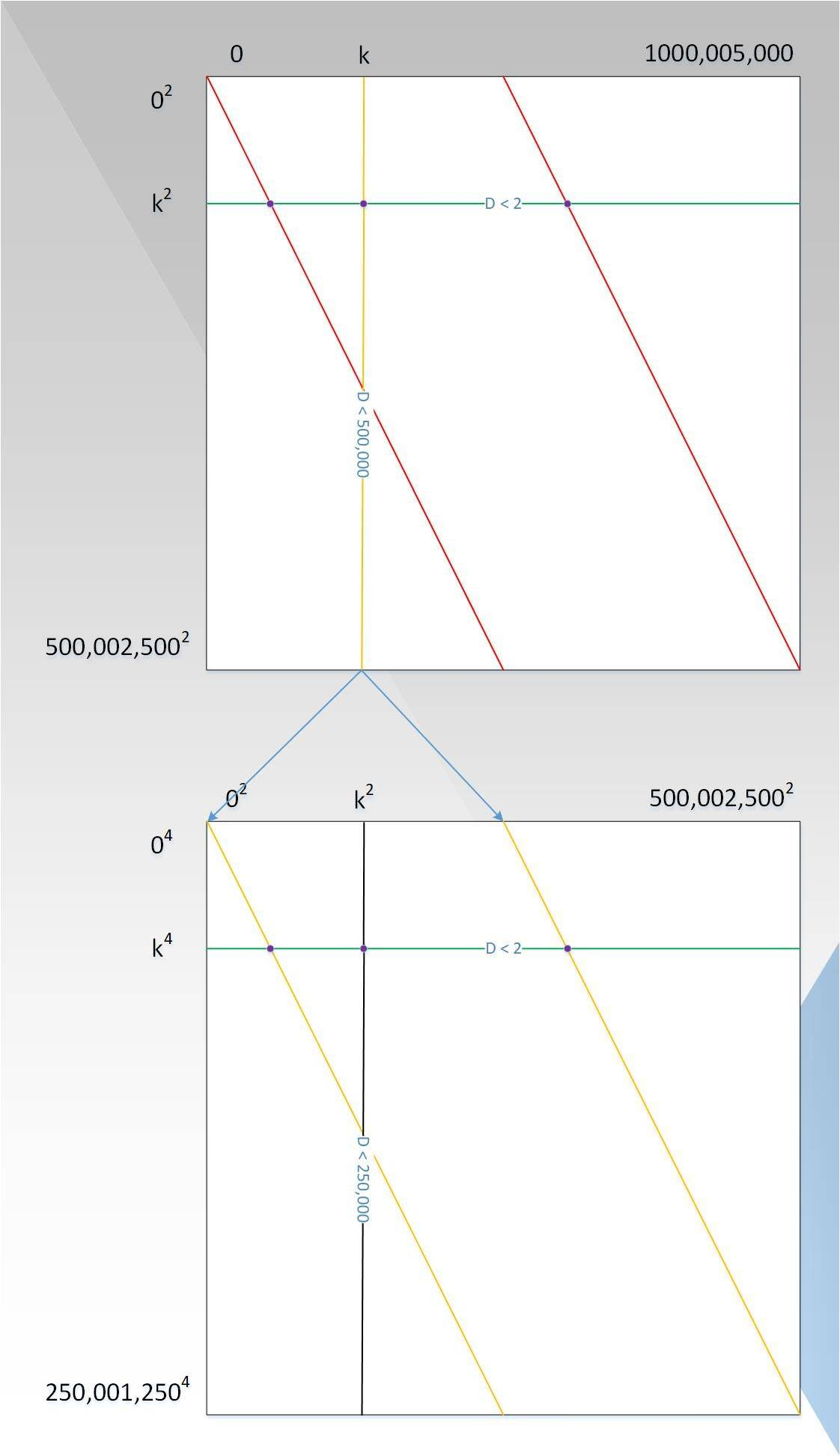• 证明则复杂度仍为 10^9 次方；
• 每一行上的点都在度 d < 2 的多项式上，因为当 y 取固定值时，G(x, y)就是关于 x 的一次多项式；
• 每一列上的点都在度 d < D/2 的多项式上，证明者需要证明这个多项式是小于 D/2 的，假定这个多项式为 P1(x)，这个时候，并非验证者选取大于 D/2 个点去验证，因为验证复杂度仍然不够低，而是对这一列再一次用到类似于 P(x) 的处理过程，如图7中下面的图所示，以此循环，直到可以直接判断列上的多项式的度为止，类似于行。

#### 总结

• 如何转换问题形式 -- Arithmetization
• 为何需要 LDT -- 为了验证简洁
• LDT 的大概过程 -- 二分法验证，类似于 FFT
• 降低 LDT 的复杂度 -- 有限域 +IOP

• 发表于 2021-02-03 11:24
• 阅读 ( 242 )
• 学分 ( 2 )
• 分类：零知识证明